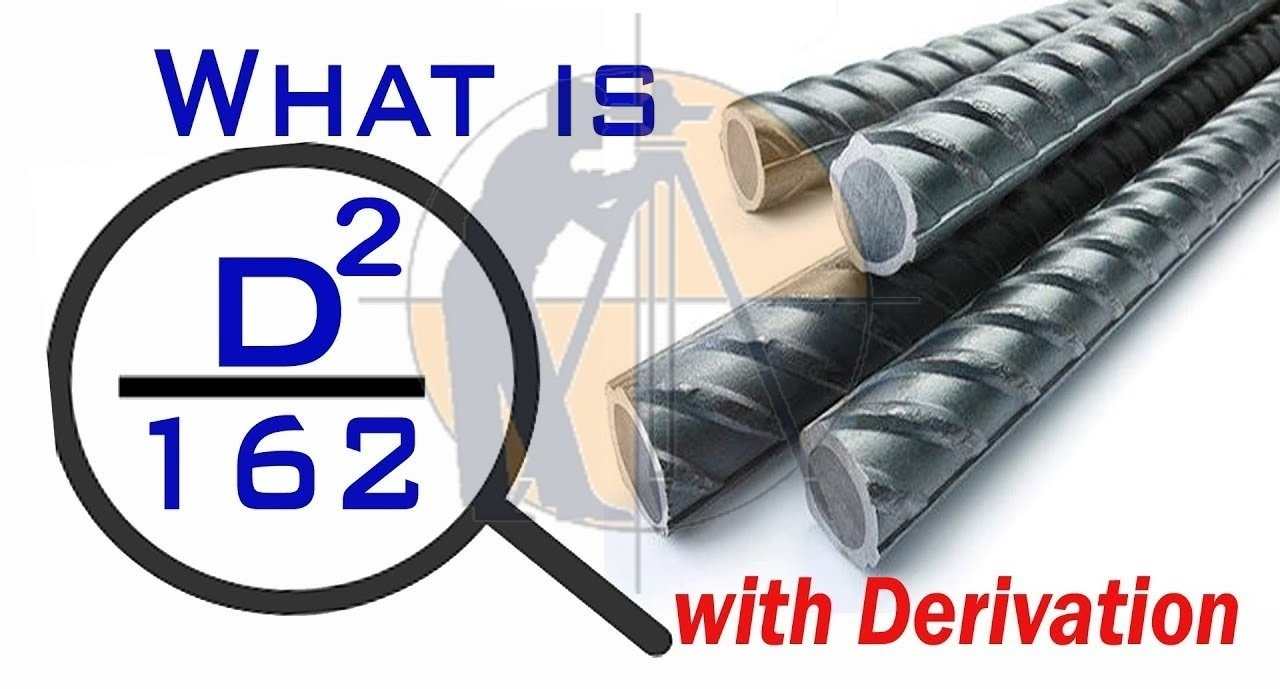Breaking News
Home / QA/QC Engineering / Unit Weight Of Steel Bar Is D2/162 & how it is derived

# Unit Weight Of Steel Bar Is D2/162 & how it is derived

## Unit Weight Of Steel Bar Is D2/162 & how it is derived

### Unit Weight of steel is D2/162 and how is it derived ?

Generally density of steel is 7850 kilogram per cubic meter and stainless steel generally vary in weight and density like 7480 to 8000 kilogram per cubic meter according to their composition. In this topic we know about weight of Steel bar  and it’s formula D square/162.

### Unit weight of steel:-

Unit Weight of steel is defined as the ratio of weight of Steel to volume of steel, Unit Weight of steel is around 7850kg/m3 or 78.5kN/m3.

### Unit Weight of Steel:-

Measured in lb./ft3 is around 490, measured in g/cm3 is around 7.85, measured in kN/m3 is around 78.5 and measured in kg/m3 is around 7850.## Why need derivation of this formula

Many of students ask to know about weight of Steel bar and its formula derivation.

And we know that for the estimate of reinforce concrete length of Steel bar is required how we know about weight of Steel bar.

## What is Unit Weight of Steel ?

Generally weight of steel is calculated in kilogram per meter. We have a formula how to calculate Unit Weight of Steel bar that is

Weight = D2L/162.

### Steel unit weight formula derivation D2L/162

Weight =volume × density

Weight= cross sectional area × length of Steel)× density of steel

Weight = πD2/4× density

### π (pai) =3.14

D=Diameter of Steel in millimeter

d = density of steel =7850kg/m3
W= weight of Steel in kilogram
L= length of Steel in meter=1meter

### Before putting all the value we should Convert millimeter into meter.

1mm =1/1000 m

Putting all the value we get

W=3.14×D2/(4×1000×1000)×1×7850 kg/m

W=D2×26649/4000000 kg/m

W=D2×0.006162 kg/m

This value we can written as follow

W= (D2/1/0.006162) kg/m

Solve 1/0.006162 =162.2

Rounding off we get 162.2~162

Weight = D2/162 kg/m

Weight = D2/162 kg/m the formula used for the unit weight calculation of Steel bar.

### Unit Weight of 8 mm,10mm,12mm,16mm,20mm Steel (rebar) length 1 meter

Weight = (8×8×1)/162= 0.395 kg/m

### *(Q2) Unit Weight of 10 mm Steel bar length 1 meter

Weight = (10×10×1)/162 =0.617 kg/m

### *(Q3) Weight of 12 mm Steel bar having length 1 meter

Weight = (12×12×1)/162 = 0.889 kg/m

### *(Q4) Weight of 16 mm Steel bar having length 1 meter

Weight = (16×16×1)/162 = 1.58 kg/m

### *(Q5) How to calculate weight of 20 mm Steel bar having length 1 meter

Weight = (20×20×1)/162 =2.469 kg/m

### THANKS.